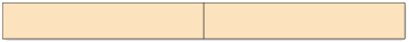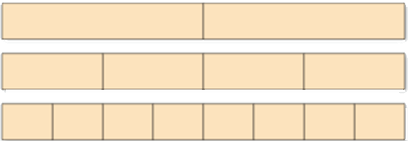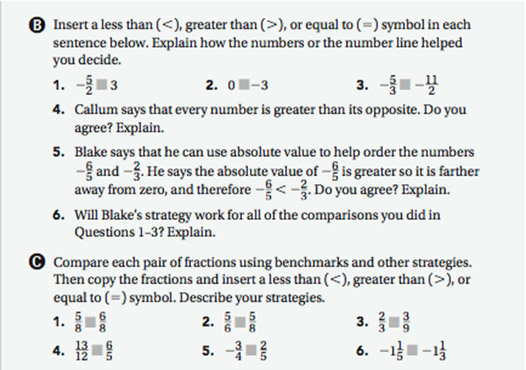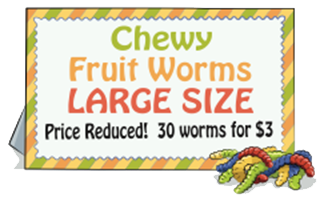Toggle Accessibility Tools

# CCSS.MATH.CONTENT.6.EE.A.1

Write and evaluate numerical expressions involving whole number exponents.

## Comparing Bits and Pieces Problem 3.3: Sharing One Hundred Things

Exponential notation is used in place value thinking required by Investigation 3 and is explicitly introduced in Problem 3.3, p. 71.

Decimals give people a way to write fractions with denominators of 10 or 100 or 1,000 or 10,000 or even 100,000,000,000, as in the table below. These denominators are different forms of base ten numeration.

Fraction Denominator as a Power of 10 Decimal
1/10 1/101 0.1
1/100 1/102 0.01
1/1,000 1/103 0.001
1/10,000 1/104 0.0001
1/100,000 1/105 0.00001
1/1,000,000 1/106 0.000001
... ... ...
1/100,000,000,000 ? ?

# CCSS.MATH.CONTENT.6.EE.A.3

Apply the properties of operations to generate equivalent expressions. For example, apply the distributive property to the expression 3 (2 + x) to produce the equivalent expression 6 + 3x; apply the distributive property to the expression 24x + 18y to produce the equivalent expression 6 (4x + 3y); apply properties of operations to y + y + y to produce the equivalent expression 3y.

Equivalence is a Big Idea in CMP3.  In Comparing Bits and Pieces, the idea of equivalence comes up again in considering equivalence of ratios, fractions, and decimals in Comparing Bits and Pieces. Here we provide three examples.

## Comparing Bits and Pieces Problem 1.2 (equivalent ratios):  Fundraising Thermometers

For a fundraiser at a middle school, each grade’s fundraising progress is recorded on charts that look like thermometers.  The thermometers are all the same length, despite the different total goals for each grade.  Each thermometer is subdivided into 10 equal parts.## Problem 1.2

A)The principal labeled some of the marks on the four thermometers with dollar amounts. Decide what lbales belong on the remaining marks.

B) 1. Ben said: For every \$60 the sixth graders plan to raise, the seventh graders plan to raise \$90. He looks at the principal's thermometers and sees that \$60 is at the same place on the sixth-grade thermometer as \$90 is on the seventh-grade thermometer.

Ben also makes the claim: For every \$30 the sixth graders plan to raise, the seventh graders plan to raise \$45.

Do you agree with this claim? Explain your reasoning.

2. Use the thermometers to write two more for every claims that relate the fundraising goals.

## A)

1. Use strips of paper 8 1/2 inchs long. Each strip represents 1 whole. Fold the strips to show halves, thirds, fourths, fifths, sixths, eighths, ninths, tenths, and twelfths. Mark the folds so you can see them easily, as shown below.2. What strategies did you use to fold your strips?

## B)

1. How can you use the halves strip to fold eighths?
2. The picture below shows a student's halves, fourths, and eighths strips. How does the size of one part of a halves strip compare to the size of one part of an eighths strip?3. What fraction strips can you make if you start with a thirds strip?
4. Which of the fraction strips you folded have at least one mark that lines up with a mark on a twelfths strip? What equilvalent fractions do the matching marks on the strips suggest?

## B)

1. Which of the fractions below coul be written with tenths or hundredths in the denominator? For each such fraction, write an equivalent decimal.
1/ 1/ 2/ 2/ 3/ 1/ 63/50  112/200
2. Which fractions cannot be written with tenths or hundredths in the denominator? Justify your answer.
1. Name two other fractions that are easy to write as equivalent decimals, and two that are not easy to write as decimals. Explain.
1. Which decimal is closest to 1/3: 0.3, 0.33 or 0.333? Explain.
2. Are any of the decimals 0.3, 0.33, 0.333 exactly 1/3? Explain your reasoning.

C) Find decimal equivalents for each group of fractions.

1. -2/5  -3/5  -4/5  -6/5
2. 2/8,  3/8,  4/8,  5/8,  6/8,  7/8
3. 1/3,  2/3,  3/3,  4/3
4. Describe the strategies you need to find decimal equivalents.

# CCSS.MATH.CONTENT.6.EE.B.5

Understand solving an equation or inequality as a process of answering a question: which values from a specified set, if any, make the equation or inequality true? Use substitution to determine whether a given number in a specified set makes an equation or inequality true.

Ordering and comparing rational numbers on a number line prepares students for later work with inequalities.

## Comparing Bits and Pieces Problem 3.2: Estimating & Ordering Rational Numbers## Comparing Bits and Pieces Problem 3.4: Decimals on the Number Line

F) Use your knowledge of fraction benchmarks and decimal place value to identify the greater number in each pair below. Use the grater than (>), less than (<), or equal to (=) symbols in writing your answers.

1. 0.1 and 0.9
2. 0.3 and 0.33
3. 0.25 and 0.250
4. 0.12 and 0.125
5. -0.1 and 0.1
6. -0.3 and -0.27

Choose three pairs of decimals from parts (1) - (6) to complete the following statements.

7. On the number line,  ? is to the left of  ? .
8. On the number line,  ?  is to the right of  ? .
9. On the number line,  ?  and  ?  share the same point.

# CCSS.MATH.CONTENT.6.EE.B.8

Write an inequality of the form x > c or x < c to represent a constraint or condition in a real-world or mathematical problem. Recognize that inequalities of the form x > c or x < c have infinitely many solutions; represent solutions of such inequalities on number line diagrams.

Thorough and explicit attention to the meaning of solving inequality equations does not formally occur until Variables and Patterns.  However, inequalities are introduced in Comparing Bits and Pieces 3.2 and 3.4 and can be extended to begin inequality reasoning.

## Comparing Bits and Pieces Problems 3.2 and 3.4 – Potential Extension

Inequalities occur in Comparing Bits and Pieces in problems 3.2 and 3.4 (see above) and offer opportunities to give some introductory attention to solving an inequality.

For instance, in Problem 3.2, Part C, Number 5 asks students to insert < or > or = symbols to complete the number sentence -3/4 - 2/5. An easy extension of such exercises might ask students, “What values of x will make the sentence x < 2/5? Draw a number line to show this comparison.”

# CCSS.MATH.CONTENT.6.EE.B.9

Use variables to represent two quantities in a real-world problem that change in relationship to one another; write an equation to express one quantity, thought of as the dependent variable, in terms of the other quantity, thought of as the independent variable. Analyze the relationship between the dependent and independent variables and graphs.

## Comparing Bits and Pieces Problem 2.3

The work with ratio in Comparing Bits and Pieces prepares students for later work with linear equations.  For instance, Problem 2.3 introduces the idea of rate table and asks students to generate sample pairs of values for related variables.A) Crystal wants to calculate costs quickly for many different numbers of chewy fruit worms. Copy and complete the rate table below with prices for each of the numbers of chewy fruit worms.

### Chewy Fruit Worm Pricing

 Number of Worms Reduced Price 1 5 10 15 30 90 150 180 \$3
1. How much do 3 chewy fruit worms cost? 300 chewy fruit worms?
2. How many chewy fruit worms can you buy for \$50? For \$10?
3. What is the unit price of one chewy fruit worm? What is the unit rate?